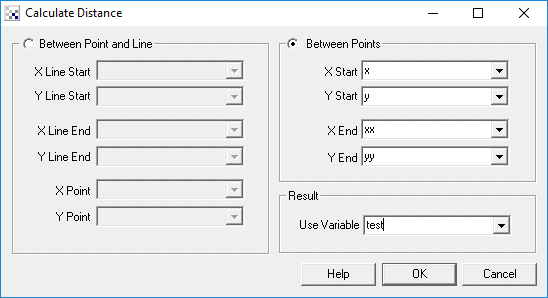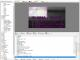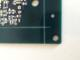# Calculate Distance

The calculate distance module provides a way to easily calculate the distance from two points. The two points are assumed to already be accessible as RoboRealm variables. The result will be placed into the result variable.

## Interface## Instructions

1. Decided on which calculate type you'd like to perform. Between points means the distance from two points, Between Point and Line means the distance from a point to a line (perpendicular distance to that line).

2. Specify the variables that contain the start and stop points of the line and/or point to measure.

3. Enter the variable that will contain the calculation result. The "Calculate_Distance" will be used if nothing is specified.

 Calculate_Distance Related Forum Posts Last post Posts ViewsProblems with Calculate_Distance and If_Statement I am surprised that I'm having difficulty with what seem to be very simple modules.  The problems are with the Calcula... 4 months 2 272Measure the distance between a hole and a line Could you outline the steps that would need to be taken, if possible, to measure the perpendicular distance from the center of t... 7 years 3 1925Calculate_distance Hi. I new in roborealm, and I have a problem. How can I calculate the distance in meters in the det... 9 years 4 2979read the distance among two colors hi, I write you from Italy, I apologize me of my a little clear English. Would I like to know if it is possible t... 10 years 15 3470 Pixels to Centimeters I want to know the position of some robots; I have a static overhead camera and use RoboRealm to acquire position. 12 years 4 12069want to calculate distance between 2 point how can i calculate a distance between 2 point.example i click the mouse at a and then click the mouse at b..then the distance a... 13 years 5 4118Calculating distance Hello, Was wondering what is the best module to be used in order to calculate the distance from the... 13 years 4 4780